# Non Verbal Reasoning - Cubes and Dice

### Exercise :: Cubes and Dice - Section 2

1.

Observe the dots on a dice (one to six dots) in the following figures. How many dots are contained on the face opposite to that containing four dots?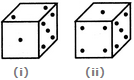A. 2 B. 3 C. 6 D. Cannot be determined

Explanation:

We shall assume the dice in fig. (ii) to be rotated so that the 5 dots appear at the same position as in fig. (i) i.e. on RHS face (i.e. on face II as per activity 1) and 1 dot appears at the same position as in fig; (i) i.e. on Front face (i.e. on face I). Then, from the, two figures, 2 dots appear on the top face (i.e. on face V) and 4 dots appear on the Bottom face (i.e. on face VI).

Since, these two faces are opposite to each other, therefore, two dots are contained on the face opposite to that containing four dots.

2.

Three different positions of a dice are shown below. How many dots lie opposite 2 dots?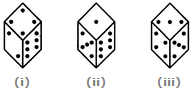A. 1 B. 3 C. 5 D. 6

Explanation:

From figures (ii) and (iii), we conclude that 1, 6, 3 and 4 dots lie adjacent to 5 dots. Therefore, 2 dots must lie opposite 5 dots. Conversely, 5 dots must lie opposite 2 dots.

3.

The six faces of a dice have been marked with alphabets A, B, C, D, E and F respectively. This dice is rolled down three times. The three positions are shown as: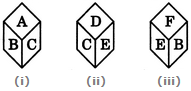Find the alphabet opposite A.

 A. C B. D C. E D. F

Explanation:

From figures (ii) and (iii), we conclude that the alphabets C, D, B and F appear adjacent to the alphabet E. Therefore, the alphabet A appears opposite E. Conversely, E appears opposite A.

4.

Three positions of a dice are given. Based on them find out which number is found opposite the number 2 in the given cube.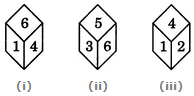A. 6 B. 5 C. 3 D. 1

Explanation:

From figures (i) and (ii), we conclude that the numbers 1, 4, 3 and 5 lie adjacent to the number 6. Clearly, the number 2 lies opposite 6 and conversely 6 lies opposite 2.

5.

A dice is thrown four times and its four different positions are shown below. Find the number on the face opposite the face showing 2.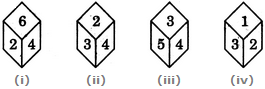A. 3 B. 4 C. 5 D. 6